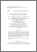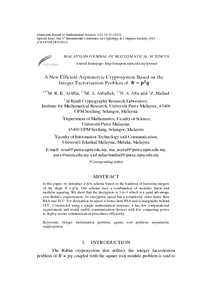# A new efficient asymmetric cryptosystem based on the integer factorization problem of N=p2q

## Citation

Kamel Ariffin, Muhammad Rezal and Asbullah, Muhammad Asyraf and Abu, Nur Azman and Mahad, Zahari (2013) A new efficient asymmetric cryptosystem based on the integer factorization problem of N=p2q. Malaysian Journal of Mathematical Sciences, 7 (S). pp. 19-37. ISSN 1823-8343; ESSN: 2289-750X

## Abstract

In this paper, we introduce a new scheme based on the hardness of factoring integers of the shape N = p2q. Our scheme uses a combination of modular linear and modular squaring. We show that the decryption is 1-to-1 which is a great advantage over Rabin's cryptosystem. Its encryption speed has a complexity order faster than RSA and ECC. For decryption its speed is better than RSA and is marginally behind ECC. Constructed using a simple mathematical structure, it has low computational requirements and would enable communication devices with low computing power to deploy secure communication procedures efficiently.Preview
PDF
39041.pdfView Item